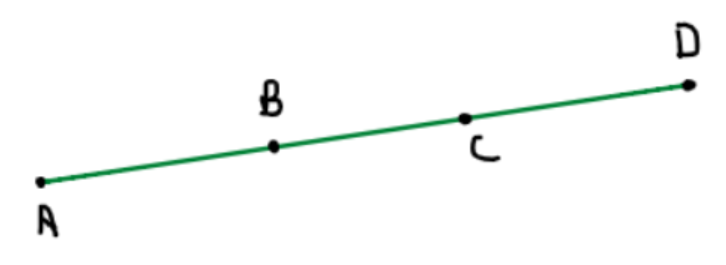Courses
Courses for Kids
Free study material
Free LIVE classes
MoreLIVE
Join Vedantu’s FREE Mastercalss

# In the figure, if AC=BD, then prove that AB=CD.Verified
364.8k+ views
Hint: Write AC as sum of 2 line segments generated by point B, similarly for BD.

It is been given in the question that
$AC = BD$……………………………… (1)
Now, it is clear from the figure that point B lies between A and C.
So, we can say that
$AC = AB + BC$…………………………….. (2)
Now, again it is clear that point C lies between B and D.
So, we can say that
$BD = BC + CD$……………………………… (3)
Now, let’s substitute equation (2) and equation (3) in equation (1) we get
$\Rightarrow AB + BC = BC + CD$
Simplifying it further, we get
$\Rightarrow AB + BC - BC = CD$
Therefore,
$AB = CD$
Hence proved.

Note - In such questions always look for the point that is dividing the line segment and write the length of the entire segment in terms of that point.

Last updated date: 25th Sep 2023
Total views: 364.8k
Views today: 3.64k